• 拉格朗日乘子法 在求取有约束条件的优化问题时，拉格朗日乘子法（Lagrange Multiplier) 和KKT条件是非常重要的两个求取方法，对于等式约束的优化问题，可以应用拉格朗日乘子法去求取最优值；如果含有不等式约束，...
拉格朗日乘子法
在求取有约束条件的优化问题时，拉格朗日乘子法（Lagrange Multiplier) 和KKT条件是非常重要的两个求取方法，对于等式约束的优化问题，可以应用拉格朗日乘子法去求取最优值；如果含有不等式约束，可以应用KKT条件去求取。当然，这两个方法求得的结果只是必要条件，只有当是凸函数的情况下，才能保证是充分必要条件。KKT条件是拉格朗日乘子法的泛化。之前学习的时候，只知道直接应用两个方法，但是却不知道为什么拉格朗日乘子法（Lagrange Multiplier) 和KKT条件能够起作用，为什么要这样去求取最优值呢？
首先把什么是拉格朗日乘子法（Lagrange Multiplier) 和KKT条件叙述一下；然后开始分别谈谈为什么要这样求最优值。
拉格朗日乘子法（Lagrange Multiplier) 和KKT条件
通常我们需要求解的最优化问题有如下几类：
(i) 无约束优化问题，可以写为
$min f(x)$;
(ii) 有等式约束的优化问题，可以写为:
​ $min f(x)$,
​ $s.t. h_i(x) = 0; i =1, …, n$
(iii) 有不等式约束的优化问题，可以写为：
​ $min f(x),$
​ $s.t. g_i(x) <= 0; i =1, …, n$
​ $h_j(x) = 0; j =1, …, m$
对于第(i)类的优化问题，常常使用的方法就是Fermat定理，即使用求取f(x)的导数，然后令其为零，可以求得候选最优值，再在这些候选值中验证；如果是凸函数，可以保证是最优解。
对于第(ii)类的优化问题，常常使用的方法就是拉格朗日乘子法（Lagrange Multiplier) ，即把等式约束h_i(x)用一个系数与f(x)写为一个式子，称为拉格朗日函数，而系数称为拉格朗日乘子。通过拉格朗日函数对各个变量求导，令其为零，可以求得候选值集合，然后验证求得最优值。
对于第(iii)类的优化问题，常常使用的方法就是KKT条件。同样地，我们把所有的等式、不等式约束与f(x)写为一个式子，也叫拉格朗日函数，系数也称拉格朗日乘子，通过一些条件，可以求出最优值的必要条件，这个条件称为KKT条件。

拉格朗日乘子法（Lagrange Multiplier)
对于等式约束，我们可以通过一个拉格朗日系数a 把等式约束和目标函数组合成为一个式子$L(a, x) = f(x) + a*h(x)$, 这里把$a和h(x)$视为向量形式，a是横向量，h(x)为列向量。
然后求取最优值，可以通过对L(a,x)对各个参数求导取零，联立等式进行求取。

KKT条件
对于含有不等式约束的优化问题，如何求取最优值呢？常用的方法是KKT条件，同样地，把所有的不等式约束、等式约束和目标函数全部写为一个式子$L(a, b, x)= f(x) + a*g(x)+b*h(x)$，KKT条件是说最优值必须满足以下条件：

$L(a, b, x)$对$x$求导为零；

$h(x) =0$;

$a*g(x) = 0$;

求取这三个等式之后就能得到候选最优值。其中第三个式子非常有趣，因为g(x)<=0，如果要满足这个等式，必须a=0或者g(x)=0. 这是SVM的很多重要性质的来源，如支持向量的概念。

有不等式约束的优化问题
假设我们求解的不等式约束的优化问题如下：
$minf(x)$
$s.t.g_i(x)⩽0 i=1,…,m$
$h_i(x)=0;i=1,…,m$
那么根据前面讲解的拉格朗日函数，我们可以得到如下的公式：
$minL(x,λ,b)=f(x)+∑^q_{i=1}λ_ig_i(x)+∑^m_{i=1}bh_i(x)$
如果我们对这样的公式求最小值会出现什么问题呢？由于没有任何的限制条件，所以λg(x)的值可能为正也可能为负，这样在无形中就增加了我们的验证难度，所以这里我们可以将$λ_i$调整为最大的正值，使λg(x)的值趋近于负无穷，使由于x变化导致的f(x)的变化基本上不会影响λg(x)的变化，这样我们就可以忽略后半部分λg(x)的值，而只关注前边f(x)的部分就可以了。这样就延伸出如下的问题：
$θp(x)=max_{λ⩾0}L(x,λ,b)$
即：求解λ最大值，使λg(x)趋近于负无穷（这个负无穷是相对的，取决于实际的情况）的值。
这样我们的问题就转变成了如下的内容：
$min_xf(x)=min_xθp(x)=min_xmax_λL(x,λ,b)$
$s.t.λ⩾0$
如果我们直接求解$min_xθp(x)$的话，不好求解，这里有两个参数，并且存在一个不等式约束，这个求解过程是比较复杂的。要求解这个过程话，我们需要先了解下拉格朗日对偶的问题。
首先思考一下，我们考虑这个公式的对偶面，即：先在λ固定的情况下，求解关于x的最小值，然后再针对λ求最大值。
如果我们只是简单的调换下求极值的顺序，那么会产生如下的结果：
$min_xmax_λL(x,λ,b)⩾max_λmin_xL(x,λ,b)$
由于我们需要的是两个等式相等，那么我们就需要寻找能够使两者相等的条件，这里呢我们就需要引入KKT条件了，KKT条件有如下几条:

∇L(x,λ,b)∇x的值为0，即拉格朗日函数对x求导的值为0。求极值的必须要求。

bi≠0。根据拉格朗日算子法，那么算子系数b的话就不能够为0（定义中的东西）。

λ⩾0。这个条件已经在前边讲过了，需要寻找不等式值的负无穷，使我们能够将求值过程简化。

$λg_i(x)=0$。

$h_i(x)=0;i=1,…,q$。这个是前提条件。

$g_i(x)⩽0;i=1,…,m$。这个也是前提条件。

拉格朗日对偶问题
如果我们求解一下不等式的优化问题：
$minf(x)$
$s.t.g_i(x)⩽0;i=1,…,m$
如果我们用KKT条件话会生成如下的函数：
$minL(x,λ)=f(x)+∑^q_{i=1}λ_ig_i(x)$
$s.t.λ_i⩾0,g_i(x)⩽0$
这个函数和我们在上面讲解的拉格朗日乘子法很想像，但是不同的是限制条件——拉格朗日算子法的限制条件是λ≠0，而KKT条件的限制条件是：λ⩾0。KKT条件实际上是对拉格朗日乘子法的泛化。
接下来将为大家展示一个基于KKT条件生成的函数(满足KTT条件)产生的一个证明过程，如下所示：
∵ $λ_i⩾0且g_i(x)⩽0$
∴ $λg(x)⩽0$
∴ $max_λL(x,λ)=f(x)$
∴ $min_xf(x)=min_xmax_λL(x,λ)$
这里我们看一下另外一个等式：
$max_λmin_xL(x,λ)=max_λ[min_xf(x)+min_xλg(x)]=max_λmin_xf(x)+max_λmin_xλg(x)=min_xf(x)+max_λmin_xλg(x) \ \ \ \ \ \ \ \ \ \ (1)$
这里讲解一下上（1）式的变化过程，对于f(x)来说，变量λ对于f(x)来说是无效的，所以我们可以进行这种变换。
$∵ λ_i⩾0且g_i(x)⩽0$
$∴λg(x)=\begin{cases}0&λ=0\ or\ g(x)=0\\−∞&λ>0\ and\ g(x)<0\end{cases}$
$∴当λ=0 或 g(x)=0时，max_λmin_xλg(x)=0∵max_λmin_xL(x,λ)=min_xf(x)+max_λmin_xλg(x)$
$∴max_λmin_xL(x,λ)=minxf(x)\ \ \ \ \ \ \ (2)$——（因为满足KTT条件是，一定有λ=0 或 g(x)=0）
据此根据（1）（2），我们可以得到如下的公式：
$max_λmin_xL(x,λ)=min_xmax_λL(x,λ)$


展开全文• 在求解最优化问题中，拉格朗日乘子法（Lagrange Multiplier）和KKT（Karush Kuhn Tucker）条件是两种最常用的方法。在有等式约束时使用拉格朗日乘子法，在有不等约束时使用KKT条件。 　我们这里提到的最优化问题...
在求解最优化问题中，拉格朗日乘子法（Lagrange Multiplier）和KKT（Karush Kuhn Tucker）条件是两种最常用的方法。在有等式约束时使用拉格朗日乘子法，在有不等约束时使用KKT条件。

我们这里提到的最优化问题通常是指对于给定的某一函数，求其在指定作用域上的全局最小值(因为最小值与最大值可以很容易转化，即最大值问题可以转化成最小值问题)。提到KKT条件一般会附带的提一下拉格朗日乘子。对学过高等数学的人来说比较拉格朗日乘子应该会有些印象。二者均是求解最优化问题的方法，不同之处在于应用的情形不同。

一般情况下，最优化问题会碰到一下三种情况：

（1）无约束条件

这是最简单的情况，解决方法通常是函数对变量求导，令求导函数等于0的点可能是极值点。将结果带回原函数进行验证即可。

（2）等式约束条件

设目标函数为f(x)，约束条件为h_k(x)，形如:

s.t. 表示subject to ，“受限于”的意思，l表示有l个约束条件。则解决方法是消元法或者拉格朗日法。消元法比较简单不在赘述，这里主要讲拉格朗日法，因为后面提到的KKT条件是对拉格朗日乘子法的一种泛化。

例如给定椭球: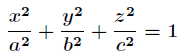求这个椭球的内接长方体的最大体积。这个问题实际上就是条件极值问题，即在条件下，求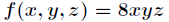的最大值。

当然这个问题实际可以先根据条件消去 z (消元法)，然后带入转化为无条件极值问题来处理。但是有时候这样做很困难，甚至是做不到的，这时候就需要用拉格朗日乘数法了。

首先定义拉格朗日函数F(x)：（ 其中λk是各个约束条件的待定系数。）

然后解变量的偏导方程：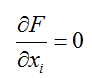......,

如果有l个约束条件，就应该有l+1个方程。求出的方程组的解就可能是最优化值（高等数学中提到的极值），将结果带回原方程验证就可得到解。

回到上面的题目，通过拉格朗日乘数法将问题转化为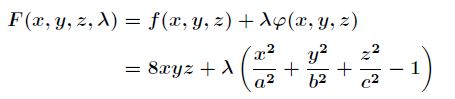对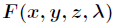求偏导得到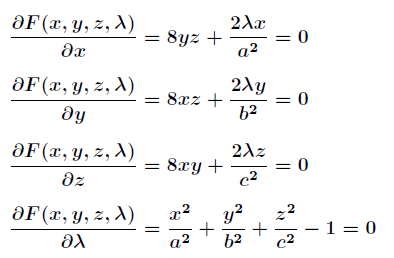联立前面三个方程得到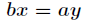和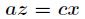，带入第四个方程解之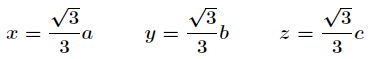带入解得最大体积为：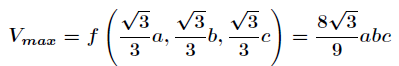（3）不等式约束条件

设目标函数f(x)，不等式约束为g(x)，有的教程还会添加上等式约束条件h(x)。此时的约束优化问题描述如下：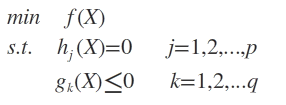则我们定义不等式约束下的拉格朗日函数L，则L表达式为：其中f(x)是原目标函数，hj(x)是第j个等式约束条件，λj是对应的约束系数，gk是不等式约束，uk是对应的约束系数。

常用的方法是KKT条件，同样地，把所有的不等式约束、等式约束和目标函数全部写为一个式子L(a, b, x)= f(x) + a*g(x)+b*h(x)，

KKT条件是说最优值必须满足以下条件：

1）L(a, b, x)对x求导为零；

2）h(x) =0;

3）a*g(x) = 0;

求取这些等式之后就能得到候选最优值。其中第三个式子非常有趣，因为g(x)<=0，如果要满足这个等式，必须a=0或者g(x)=0. 这是SVM的很多重要性质的来源，如支持向量的概念。

接下来主要介绍KKT条件，推导及应用。详细推导过程如下：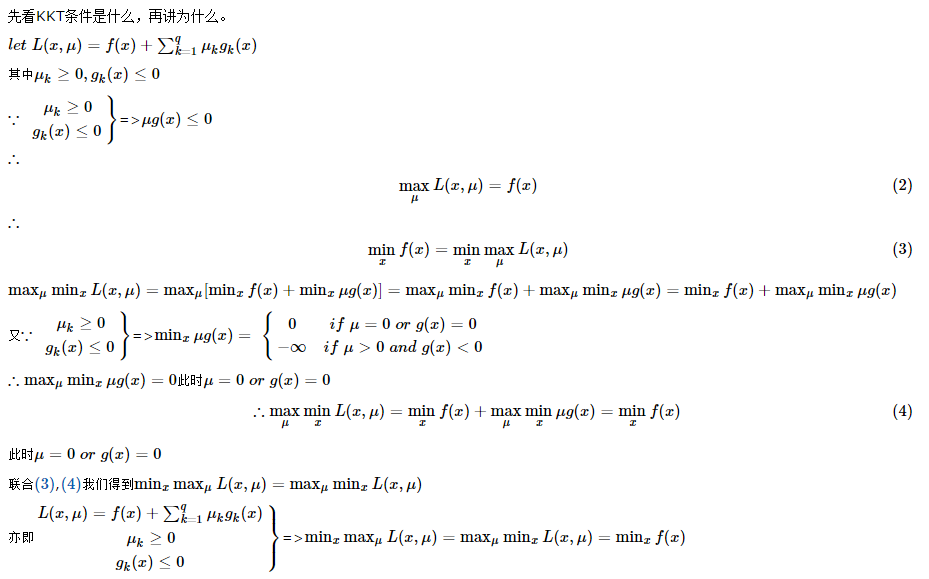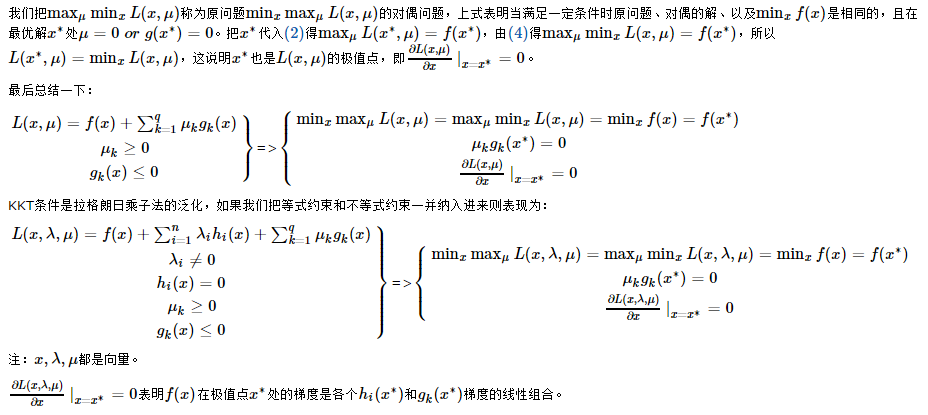？？？？从几何角度看拉格朗日乘子法的物理意义：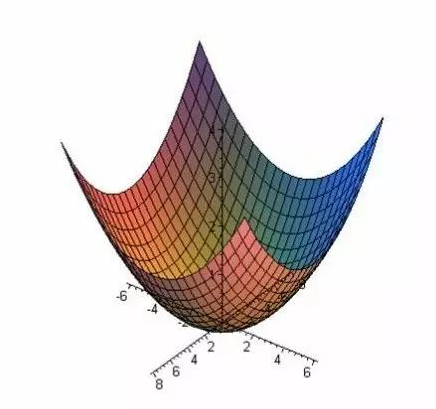该方法适用于约束条件下求极值的问题。对于没有约束的极值问题，显然，如果某一点是极值的必要条件是该点的各方向的偏导数皆为零，也就是说，如果偏导数不全为零，那么就不可能是极值。

例如，一个三元函数w(x,y,z), 它是x,y,z的函数，且在一个约束条件下求它的极值。我们假设图中的曲面就是约束方程g(x,y,z)=0的图像，即约束面。之前没有约束面时，w取极值的必要条件是各个方向偏导数为零，而对于可微函数各个方向偏导为零的充分必要条件是沿x,y,z 方向的偏导为零。现在有了约束面，我们不再需要这么苛刻的必要条件，因为有了约束面，x,y,z在一定程度上被限制了，只能在约束面内移动，因此只需要沿约束面内的各个方向运动时的偏导数（变化化率）为零就可以了，此时自由度由三维下降到两维。满足在约束面内的各个方向偏导为零，也就是说，w取极值的必要条件减弱为待求函数的方向导数（梯度）垂直于约束面，从数学上看，也就是方向导数和约束面的法线方向同向（一个向量等于另一个向量的常数倍），而不需要梯度为零，因为和梯度垂直的方向偏导数一定为零，这样，沿约束面各个方向运动时w的偏导数也就为零了。这便是拉格朗日乘子法求极值的几何意义。

个人总结：

想象一下我们爬山（优化函数）找最高点（求最大值），要想最快的上，要找最陡的方向，陡峭的程度以坡度（方向导数）度量，最陡的方向即为最大坡度（梯度）决定的方向，理想情况下，当无法再上升，坡度（梯度）变成0时，找到最高点（求得最大值）。但是，当我们必须绕圆弧行盘山路爬行时，盘山路（约束条件）约束了我们的路径及方向，我们必须沿着盘山路最陡的方向（梯度，注意此时退化为一维，只有一个方向，为道路切向），当道路不再上升（及切向为0），即找到最高点。

再想想一下我们是海水，从山底向上移动（集体作战），领袖沿着盘山路行进，每一步我们可以找到同海拔的海岸线（等高线），海岸线与盘山路想交，我们可以继续向上移动，直到海岸线与盘山路向切，此时，找到最高海拔，海岸线（等高线）同时与约束方程确定的边界相切。

在极值点，优化函数的等高线、优化函数与约束方程的交线、约束方程的投影线（类似约束曲面的等高线，约束曲线）相切于一点。等高线与约束曲线法向相同（不考虑正负），而优化函数的梯度数值等于其等高线的法向数值，约束方程的梯度数值等于约束曲线的法向数值。故∆f=λ∆g,λ!=0

极值点的2个条件：

1、极值点在优化函数及约束方程上；

2、在极值点，优化函数的等高线、优化函数与约束方程交线、约束曲线相切，优化函数与约束方程交线的梯度（导数）为0

可利用这2个条件求解：

一、根据1将约束方程带入优化函数消元、降维变成无约束低维问题求解，根据2求梯度为0

二、根据2构造似然函数L（X，λ），使在特殊条件下满足1和2，对L（X,λ）解特殊条件。


展开全文数学 KKT条件
• 在SVM中，将约束问题转化成非约束问题采用到了拉格朗日乘子法。这个文章就讲一下拉格朗日乘子法与KKT约束是怎么回事。本人不是数学科班出身，但是也只能硬着头皮讲一讲了。从零理解现在我们要解决这样一个问题：这个...在SVM中，将约束问题转化成非约束问题采用到了拉格朗日乘子法。这个文章就讲一下拉格朗日乘子法与KKT约束是怎么回事。本人不是数学科班出身，但是也只能硬着头皮讲一讲了。从零理解现在我们要解决这样一个问题：这个函数距离原点最近的距离是多少。先画出函数图像：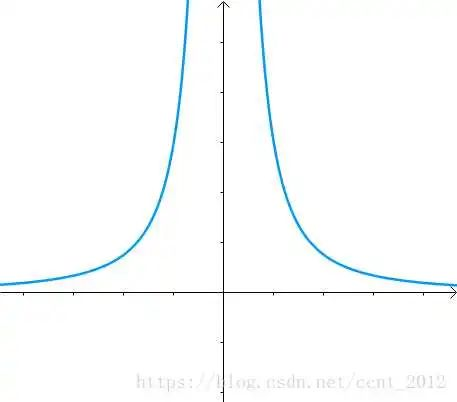然后想求出最短距离：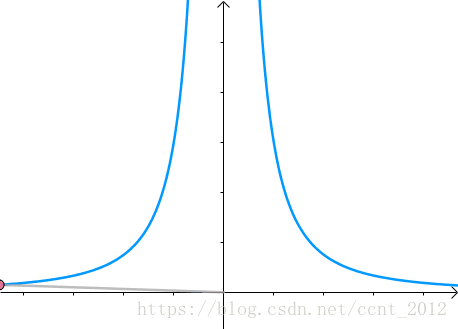这里的思路就是，做一个以原点为中心的圆形：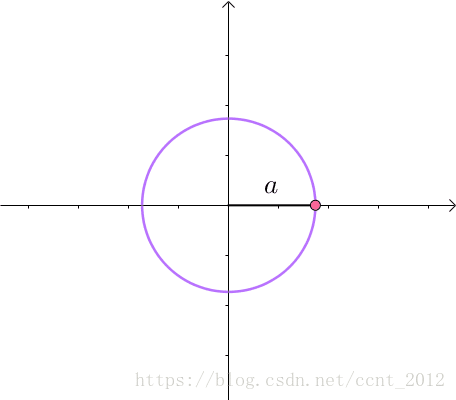不断扩大圆形的半径，直到圆与蓝色的曲线相切：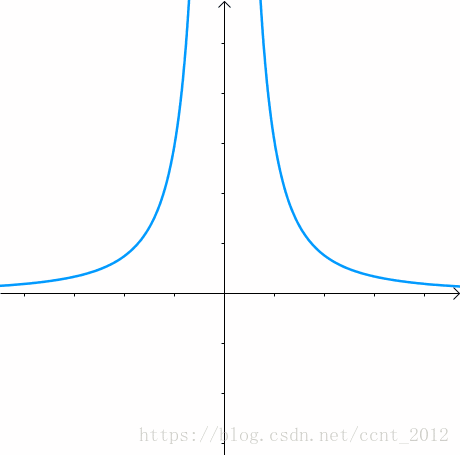现在。第一次与相交的点就是距离原点最近的那个点：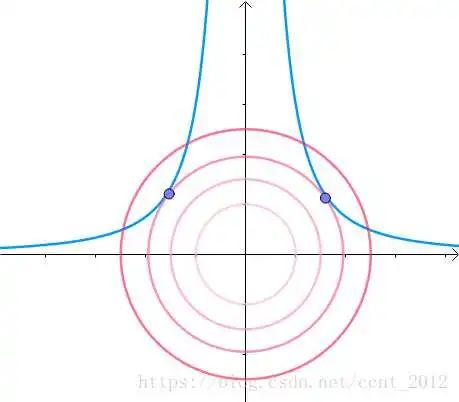这个，圆形与曲线相切，且切线既是圆形的切线，也是曲线的相切。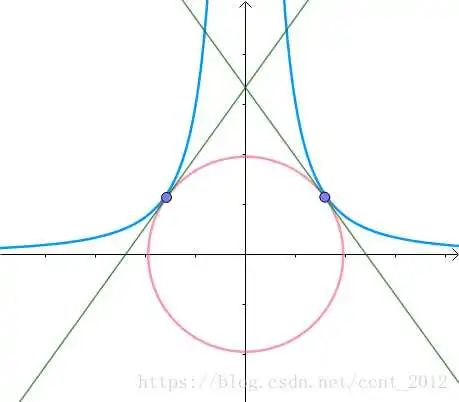这时候，这个切线的垂线其实也就是我们所说的梯度，也叫做等高线的法线，看下面两个图可能会好理解一些：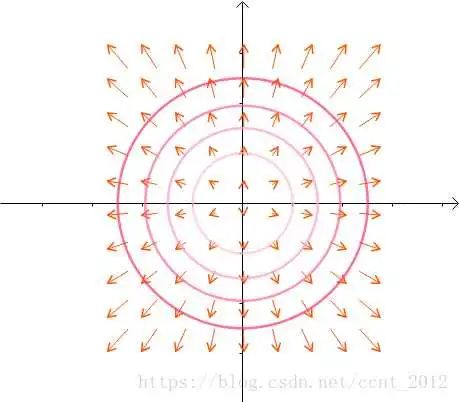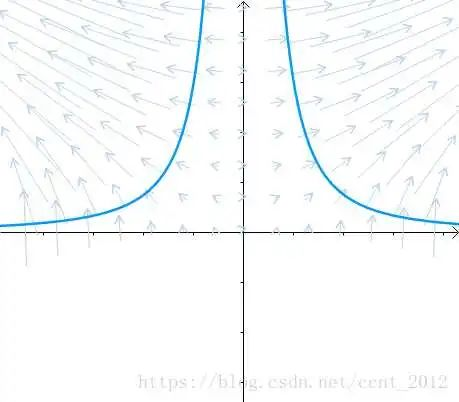那么这个梯度怎么计算呢？先看圆形的梯度：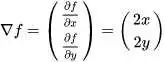再看曲线的梯度计算的梯度：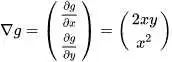在相切的时候，两者的梯度方向都在同一条直线上，可以称之为，成比例，这里用比例系数来表示：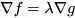所以我们汇总一下所有的已知信息，得到下面的方程组：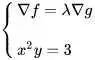可以求解得到：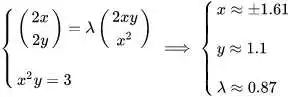这个就是拉格朗日乘子法的直观理解。抽象成数学的形式我们要解决的问题：我们会将约束问题通过拉格朗日乘子法转换成非约束问题：【为什么可以这样呢？】如果求极值，偏导数为0。先对上面的公式进行求偏导数：这两个等式与这个等价，唯一的不同就是一个是正数一个是负数: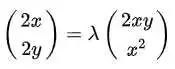当然，对于这个条件，我们也可以写成，所以，可以得到这样的一个方程组：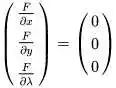KKT条件KKT的英文全称：Karush-Kuhn-Tucker之前的拉格朗日的约束条件是等值的，现在可以通过KKT条件推广到不等式。因为限制条件往往是不大于，小于这样的不等式，所以KKT才是拉格朗日化约束问题为非约束问题的关键。对于不等式问题，就是有两种情况：可行解在g(x)<0；可行解在g(x)=0。可行解在g(x)<0，就表示这个约束条件并没有起到约束效果，有跟没有是一个效果(下图中的左图)；可行解g(x)=0,就表示这个约束条件起到作用了，这就表示g(x)与f(x)相切，也就是下图中右边的图。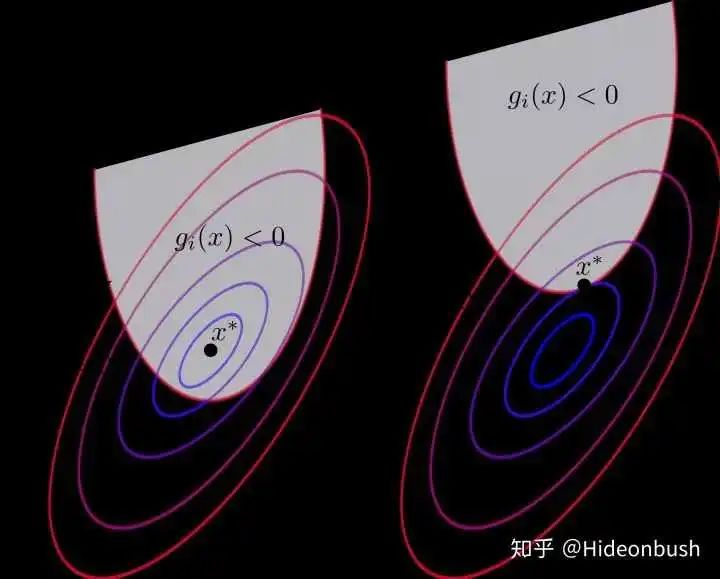【g(x)<0的情况】这种情况下，就是没有限制条件下的情况，其实就是没有约束条件的限制，也就是的情况，所以我们的等式就是直接求解：【g(x)=0的情况】如果是g(x)=0的情况，那也就是约束条件起到作用了，也就意味着。在这种情况下，存在着:并且两个函数的扩张的方向相反，所以表明两个g(x)和f(x)的梯度一个是正数，一个是负数。所以这个表示。所以综上所述，在这种情况下，我们所有的条件综合起来可以得到，其中就是最优解：这三个就是KKT条件。<>项目总结 | 八种缺失值处理方法总有一种适合你项目总结 | 对 "时间" 构建的特征工程AI面试题之SVM推导AI面试扩展之LightGBM = GOSS + histogram + EFBAI面试题之XGBoost与手推二阶导AI面试题之GBDT梯度提升树AI面试题之防止过拟合的所有方法AI面试题之梯度消失(爆炸)及其解决方法<> 大汇总 | 一文学会八篇经典CNN论文一分钟速学 | NMS, IOU 与 SoftMax图像增强 | CLAHE 限制对比度自适应直方图均衡化干货 | BatchNormalization详解与比较【评价指标】详解F1-score与多分类MacroF1&MicroF1AI面试题之(反)卷积输出尺寸计算强烈推荐 | 李宏毅老师“2020年最新深度学习从入门到精通视频教程”，公众号回复【李宏毅老师】免费获取~关注公众号，回复【下载】有免费的精选的机器学习相关的PDF学习资料，持续更新哦！回复【入群】，加入校招微信群，和大佬一起交流面试心得。公众号经常会有福利送书活动哦~展开全文• ﻿前言本文主要对拉格朗日乘子法进行总结，具体原理可以参考这两篇文章：友情链接 1友情链接 2拉格朗日乘子法基本的拉格朗日乘子法主要是针对等式约束下的非线性方程最优化问题，具体形式如下： 对于上述问题，我们...
﻿前言本文主要对拉格朗日乘子法进行总结，具体原理可以参考这两篇文章：友情链接 1友情链接 2拉格朗日乘子法基本的拉格朗日乘子法主要是针对等式约束下的非线性方程最优化问题，具体形式如下：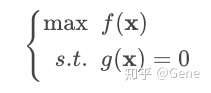对于上述问题，我们可以构造拉格朗日算符函数，然后可以得到最优解的必要条件如下：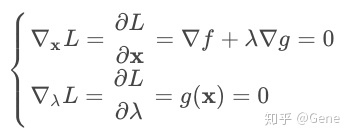Karush-Kuhn-Tucker (KKT) 条件我们可以将上述的等式约束推广到不等式约束下，依然可以有方法进行解决，具体形式如下：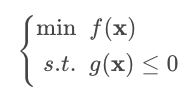对于上述问题，我们依然是构造拉格朗日算符函数，但是最优解的条件发生了改变，具体条件如下：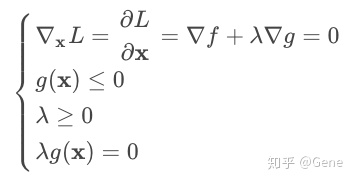上述条件即为条件，接下来我们给出非线性规划的标准形式：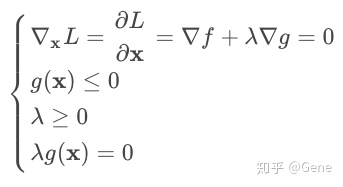对于上述标准形式的非线性规划问题，我们可以定义拉格朗日算符函数，其中对应等式约束的拉格朗日乘数，对应不等式约束的拉格朗日乘数。由此我们可以综合上述等式约束与不等式约束的最优值必要条件，具体如下：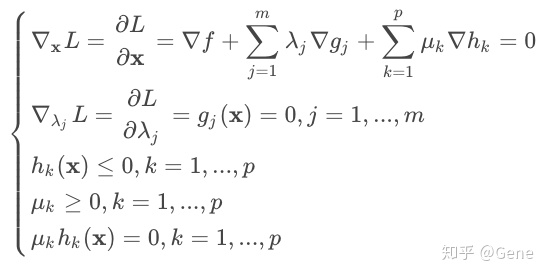接下来我们以一道习题为例来进行讲解。2020牛客暑期多校训练营（第一场）D.Quadratic Form题意给定一个的正定对称矩阵以及一个维向量，想要求解如下非线性规划问题：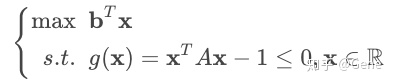，最终答案。思路很明显，这是一道基于条件的问题。由于本问题是求，因此我们需要转换成求的最小值，构造拉格朗日算符函数如下：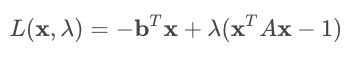接下来我们可以得到如下的条件，即取到极值的必要条件：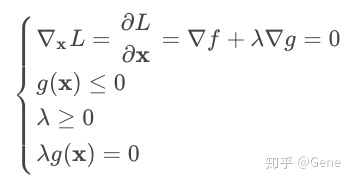由此可以得到如下两条等式：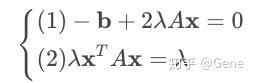化简式，可以得到，代入式可以得到，注意，继续化简式如下：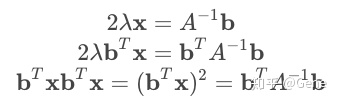因此本题的最终答案为，只需套入矩阵求逆的板子即可解决。代码#include <bits/stdc++.h>
#define rep(i,a,b) for(int i = a; i <= b; i++)
typedef long long ll;
const int N = 405;
const ll mod = 998244353;
using namespace std;

ll A[N][N], b[N], ans[N];
int n, B1[N], B2[N];

ll poww(ll a, ll b) {
ll base = a, ans = 1;
while(b) {
if(b & 1) ans = ans * base % mod;
base = base * base % mod;
b >>= 1;
}
return ans;
}

bool matrixInv() {
for(int k = 1; k <= n; k++) {
for(int i = k; i <= n; i++)
for(int j = k; j <= n; j++)
if(A[i][j]) {
B1[k] = i, B2[k] = j; break;
}
for(int i = 1; i <= n; i++)
swap(A[k][i], A[B1[k]][i]);
for(int i = 1; i <= n; i++)
swap(A[i][k], A[i][B2[k]]);
if(!A[k][k]) {
return false; // 不可逆
}
A[k][k] = poww(A[k][k], mod-2);
for(int j = 1; j <= n; j++)
if(j!=k) (A[k][j] *= A[k][k]) %= mod;
for(int i = 1; i <= n; i++)
if(i != k)
for(int j = 1; j <= n; j++)
if(j != k)
(A[i][j] += mod - A[i][k] * A[k][j] %mod) %= mod;
for(int i = 1; i <= n; i++)
if(i != k)
A[i][k] = (mod - A[i][k] * A[k][k] % mod) % mod;
}
for(int k = n; k; k--) {
for(int i = 1; i <= n; i++)
swap(A[B2[k]][i], A[k][i]);
for(int i = 1; i <= n; i++)
swap(A[i][B1[k]], A[i][k]);
}
return true; // 可逆
}

int main()
{
while(~scanf("%d",&n)) {
rep(i,1,n)
rep(j,1,n) scanf("%lld",&A[i][j]);
rep(i,1,n) scanf("%lld", &b[i]);
matrixInv();
rep(i,1,n) {
ll v = 0;
rep(j,1,n) v = (v + b[j] * A[i][j]) % mod;
ans[i] = v;
}
ll res = 0;
rep(i,1,n) res = (res + ans[i] * b[i]) % mod;
printf("%lldn", res);
}
return 0;
}

展开全文• 在此之前，我对优化理论都没有很好地理解，今天看了一些知乎上大神们的解释，对拉格朗日乘子法和KKT条件有了一些全新的认识，今天特意mark一下。感谢https://zhuanlan.zhihu.com/p/36621652​zhuanlan.zhihu.com和非...
• 什么是拉格朗日乘子法？在数学最优问题中，拉格朗日乘子法（Lagrange Multiplier，以数学家拉格朗日命名）是一种寻找变量受一个或多个条件限制的多元函数的极值的方法。这种方法将一个有n 个变量与k 个约束条件的最...
• 今天碰到一个问题， 这个函数，因为-log(x)凸函数的性质，我们可以知道是大于等于log（N）的。或者可以解释为相对熵plog(p/q)总是大于0的。其实这一点在信息论里面也有...可以利用拉格朗日乘子法对这个函数的最小值...
• 拉格朗日乘子法大家都学过，用来求带约束条件的极制问题，用起来着实简单，只是上学的时候只学会怎么用了，到底为什么这样能行是从来没想过的。最近在看B站机器学习课程，看到SVM这一段，用到拉格朗日乘子法，老师讲......